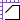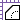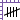# Measure Toolbar

By default, the Measure toolbar provides ready access to the Measure and Calibrate tools as well as the measurement modes. Tools commonly found on this toolbar are described below. The exact tools a user will see depends on their Profile and might not include all the tools mentioned here. For a complete list of tools that can be placed on a toolbar and more information about customizing them, see Tools and Toolbars.If the toolbar is currently hidden, go to Tools > Toolbars and select it to show it.Measure (M): Launches the Measure tool and opens the Measurements panel.Set Scale: Sets the document's scale using one of several methods to allow accurate measurements to be taken.Length (SHIFT+ALT+L): Calculates a singular linear measurement.Polylength (SHIFT+ALT+Q): Creates specialized markups that calculate multiple, linear measurements as well as their cumulative length.Perimeter (SHIFT+ALT+P): Calculates the length of all the sides of a given shape or multi-segment line.Area (SHIFT+ALT+A): Calculates the area of a simple rectangle or of an irregular, polygonal shape.Volume (SHIFT+ALT+V): Calculates the volume of an area with a defined depth.Polygon Cutout: Removes a polygonal area from an existing Area or Volume measurement.Ellipse Cutout: Removes an elliptical area from an existing Area or Volume measurement.Dynamic Fill (J): Automatically creates Spaces and measurement markups from any shape on the content layer of a drawing.Count (SHIFT+ALT+C): Places a markup for each mouse click, associating a running total of counts with each markup as it is placed.Diameter (SHIFT+ALT+D): Calculates the diameter of a circular shape.Angle (SHIFT+ALT+G): Measures a given angle as defined by three points.Radius (SHIFT+ALT+U): Measures the radius a circle, defined either center-out or by indicating any three points along its circumference.